suppressPackageStartupMessages(library(tidyverse))
library(gapminder)
knitr::opts_chunk\$set(fig.width=5, fig.height=3)

From this lecture, students are expected to be able to:

• Describe the scatterplot of an independent bivariate Gaussian/Normal distribution.
• Describe whether there’s dependence, given a normal scores plot of the data.

## Question

What is data science?

## Dependence

Concepts:

• Dependence is the absence of independence, and is more than just linear association.
• When asking for an “amount of dependence”, indicators measure how tightly the points adhere to a curve.
• You can’t add/remove dependence through monotone transformations.
• Dependence is more than just linear association.
• Dependence helps us get information of one variable if we know something about the other.
• Normal scores plots are useful for viewing dependence unobscured by the marginals.

### Independence demo

Gaussian demo: what does independent bivariate Guassian look like? Let’s try different mean and sd’s.

n <- 100
x <- rnorm(n)
y <- rnorm(n)
qplot(x, y)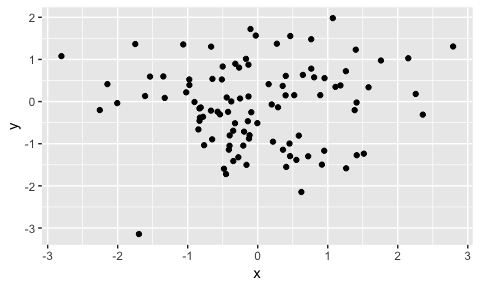Generic independence:

1. Give me a marginal distribution for x, and one for y.
2. View a scatterplot.
3. Try monotone transformations on x or y to try to make dependence.
n <- 100
# x <-
# y <-
qplot(x, y)Practicalities of independence: x and y do not inform each other.

### Dependence demo

How do you answer the question “how much dependence is there?”

Demo:

1. Give me a way in which x and y can depend on each other.
2. View a scatterplot.
3. Try monotone transformations on x or y. Does the “amount” of dependence change?
n <-
# x <-
# y <-
qplot(x, y)

Practicalities of dependence: each variable informs the other. Useful if we know something about one, and want to know something about the other (this is a HUGE part of data science!)

### Normal Scores Plots

Normal scores plots are a useful way to standardize the viewing of dependence (amongst two variables).

1. Transform margins to N(0,1):
1. First transform to uniform scores, by dividing the rank by n.
2. Then, apply qnorm().
2. Make the scatterplot of the transformed variables.

Function for Step 1:

nscore <- function(x) qnorm(ecdf(x)(x))

Convert the following scatterplot to a normal scores plot. Is there dependence?

n <- 1000
x <- rexp(n)
y <- rexp(n)
qplot(x, y)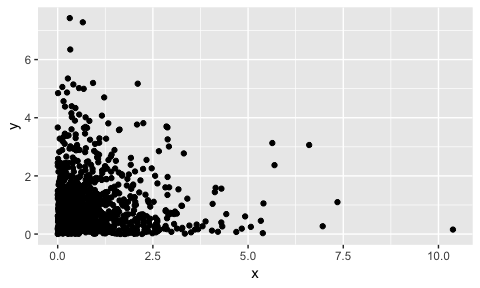Try making a normal scores plot of previous examples OR data examples:

EDA is not about fishing for meaning. Ask yourself this question: what does this graph show that we can’t see from viewing the raw data set?

Examples:

ggplot(mtcars, aes(disp, carb)) +
geom_point(alpha=1/3) +
labs(x = "Displacement",
y = "Number of carburators")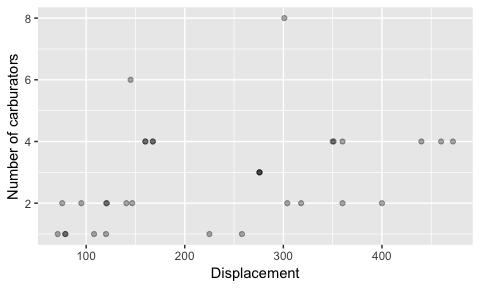Nothing going on in the above plot. End of story.

mtcars %>%
mutate(cyl = paste0(cyl, "-cylinder")) %>%
ggplot(aes(wt, hp)) +
geom_point() +
facet_wrap(~ cyl)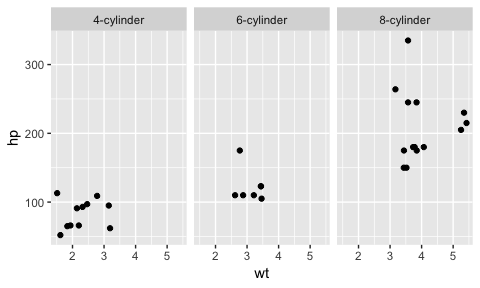Nothing going on within each panel above. Though, more cylinders tend to have higher hp.

ggplot(iris, aes(Sepal.Width, Petal.Width)) +
geom_jitter()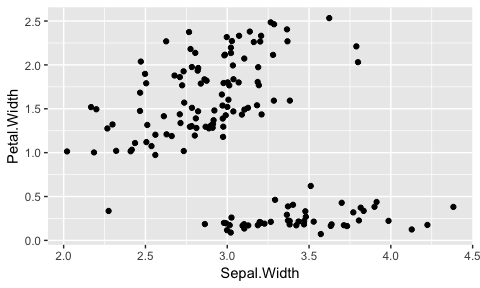Data fall into at least two groups. Explore further:

ggplot(iris, aes(Sepal.Width, Petal.Width)) +
geom_jitter(aes(colour=Species))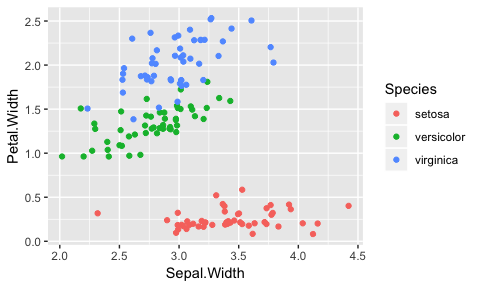Data fall into three groups! Is there dependence within each group? Check against normal scores plot:

ggplot(iris, aes(nscore(Sepal.Width), nscore(Petal.Width))) +
geom_jitter() +
facet_wrap(~ Species, scales="free")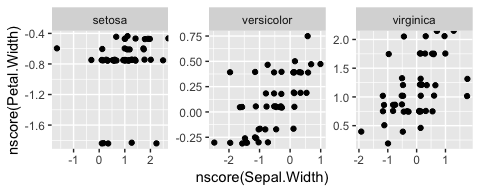Not much. Maybe some positive dependence in versicolor.

## Worksheet

Let’s fill out the worksheet on the remaining ggplot2 tooling.